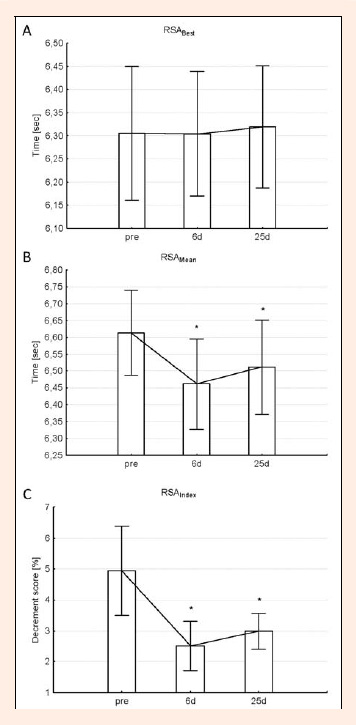Figure 3. Changes in best sprint time (Repeated-Sprint Ability (RSA)) (a) Cohen’s d (pre-6d) = 0.07; Cohen’s d (6d-25d) = 0.15, mean sprint time (Repeated-Sprint Ability (RSA)) (b) Cohen’s d (pre-6d) = 1.15; Cohen’s d (6d-25d) = 0.37 and the fatigue index (Repeated-Sprint Ability (RSA)) (c) Cohen’s d (pre-6d) = 1.99; Cohen’s d (6d-25d) = 0.7. * = significantly different to pre (p < 0.05). Values are presented as mean ± SD.